UIHistories Project: A History of the University of Illinois by Kalev Leetaru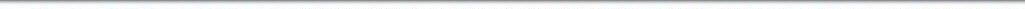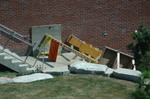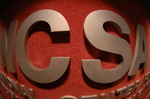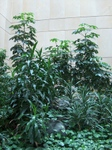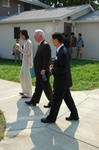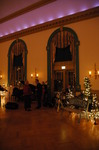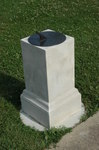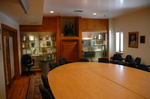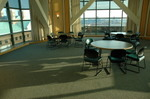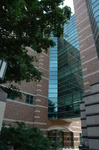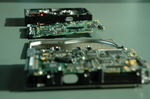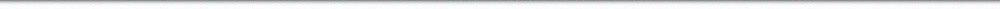N A V I G A T I O N D I G I T A L L I B R A R Y

## Repository: UIHistories Project: Board of Trustees Minutes - 1896 [PAGE 179]

Caption: Board of Trustees Minutes - 1896
This is a reduced-resolution page image for fast online browsing.

< Previous Page [Displaying Page 179 of 371] Next Page >
[VIEW ALL PAGE THUMBNAILS]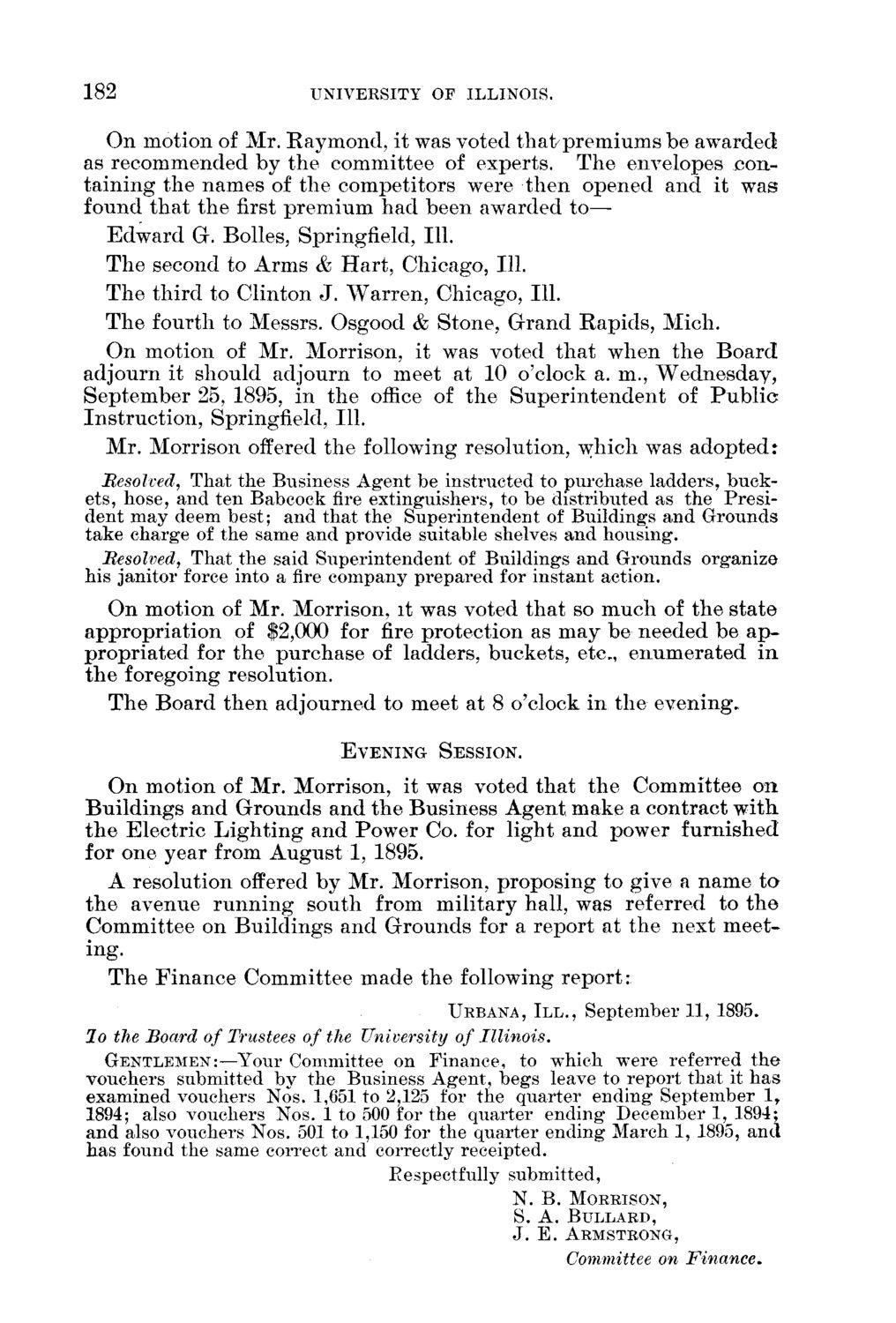### EXTRACTED TEXT FROM PAGE:

182

UNIVERSITY OF ILLINOIS.

O n motion of M r . R a y m o n d , it was voted t h a t / p r e m i u m s b e awarded as r e c o m m e n d e d b y t h e c o m m i t t e e of experts. T h e envelopes c o n t a i n i n g t h e n a m e s of t h e c o m p e t i t o r s were t h e n opened a n d it w a s found t h a t t h e first p r e m i u m h a d b e e n awarded t o — E d w a r d G. Bolles, Springfield, 111. T h e second t o A r m s & H a r t , Chicago, 111. T h e t h i r d to C l i n t o n J . W a r r e n , Chicago, 111. T h e fourth to Messrs. Osgood & S t o n e , G r a n d R a p i d s , M i c h . O n motion of M r . Morrison, it was voted t h a t w h e n t h e B o a r d adjourn it should adjourn to meet at 10 o'clock a. m., W e d n e s d a y , S e p t e m b e r 25, 1895, i n t h e office of t h e S u p e r i n t e n d e n t of P u b l i c I n s t r u c t i o n , Springfield, 111. M r . M o r r i s o n offered t h e following resolution, w h i c h was a d o p t e d : Besolvedy That the Business Agent be instructed to purchase ladders, buckets, hose, and ten Babcock fire extinguishers, to be distributed as the President may deem best; and that the Superintendent of Buildings and Grounds take charge of the same and provide suitable shelves and housing. Resolved, That the said Superintendent of Buildings and Grounds organize his janitor force into a fire company prepared for instant action. O n m o t i o n of M r . Morrison, it was voted t h a t so m u c h of t h e s t a t e a p p r o p r i a t i o n of \$2,000 for fire p r o t e c t i o n as m a y b e n e e d e d b e a p p r o p r i a t e d for t h e p u r c h a s e of ladders, buckets, etc., e n u m e r a t e d i n t h e foregoing resolution. T h e B o a r d t h e n adjourned to meet at 8 o'clock i n t h e e v e n i n g .

EVENING SESSION.

O n m o t i o n of M r . Morrison, it was voted t h a t t h e C o m m i t t e e o n B u i l d i n g s a n d G r o u n d s a n d t h e B u s i n e s s A g e n t m a k e a c o n t r a c t with, t h e E l e c t r i c L i g h t i n g a n d P o w e r Co. for light a n d power f u r n i s h e d for one year from A u g u s t 1, 1895. A resolution offered b y M r . Morrison, p r o p o s i n g to give a n a m e t o t h e a v e n u e r u n n i n g s o u t h from m i l i t a r y hall, was referred t o t h e C o m m i t t e e on B u i l d i n g s a n d G r o u n d s for a r e p o r t at t h e n e x t m e e t ing. T h e F i n a n c e C o m m i t t e e m a d e t h e following r e p o r t :

URBANA, I I I . , September 11, 1895.

lo the Board of Trustees of the University of Illinois. GENTLEMEN:—Your Committee on Finance, to which were referred the vouchers submitted by the Business Agent, begs leave to report that it has examined vouchers Nos. 1,651 to 2,125 for the quarter ending September l r 1894; also vouchers Nos. 1 to 500 for the quarter ending December 1, 1894; and also vouchers Nos. 501 to 1,150 for the quarter ending March 1, 1895, and has found the same correct and correctly receipted. Eespectfully submitted,

N. B. MORRISON, S. A. BULLARD, J . E. ARMSTRONG,

Committee on Finance.